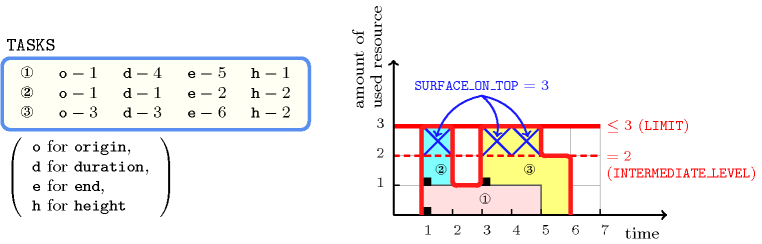## 5.361. soft_cumulative

Origin
Constraint

$\mathrm{𝚜𝚘𝚏𝚝}_\mathrm{𝚌𝚞𝚖𝚞𝚕𝚊𝚝𝚒𝚟𝚎}\left(\mathrm{𝚃𝙰𝚂𝙺𝚂},\mathrm{𝙻𝙸𝙼𝙸𝚃},\mathrm{𝙸𝙽𝚃𝙴𝚁𝙼𝙴𝙳𝙸𝙰𝚃𝙴}_\mathrm{𝙻𝙴𝚅𝙴𝙻},\mathrm{𝚂𝚄𝚁𝙵𝙰𝙲𝙴}_\mathrm{𝙾𝙽}_\mathrm{𝚃𝙾𝙿}\right)$

Arguments
 $\mathrm{𝚃𝙰𝚂𝙺𝚂}$ $\mathrm{𝚌𝚘𝚕𝚕𝚎𝚌𝚝𝚒𝚘𝚗}\left(\begin{array}{c}\mathrm{𝚘𝚛𝚒𝚐𝚒𝚗}-\mathrm{𝚍𝚟𝚊𝚛},\hfill \\ \mathrm{𝚍𝚞𝚛𝚊𝚝𝚒𝚘𝚗}-\mathrm{𝚍𝚟𝚊𝚛},\hfill \\ \mathrm{𝚎𝚗𝚍}-\mathrm{𝚍𝚟𝚊𝚛},\hfill \\ \mathrm{𝚑𝚎𝚒𝚐𝚑𝚝}-\mathrm{𝚍𝚟𝚊𝚛}\hfill \end{array}\right)$ $\mathrm{𝙻𝙸𝙼𝙸𝚃}$ $\mathrm{𝚒𝚗𝚝}$ $\mathrm{𝙸𝙽𝚃𝙴𝚁𝙼𝙴𝙳𝙸𝙰𝚃𝙴}_\mathrm{𝙻𝙴𝚅𝙴𝙻}$ $\mathrm{𝚒𝚗𝚝}$ $\mathrm{𝚂𝚄𝚁𝙵𝙰𝙲𝙴}_\mathrm{𝙾𝙽}_\mathrm{𝚃𝙾𝙿}$ $\mathrm{𝚍𝚟𝚊𝚛}$
Restrictions
 $\mathrm{𝚛𝚎𝚚𝚞𝚒𝚛𝚎}_\mathrm{𝚊𝚝}_\mathrm{𝚕𝚎𝚊𝚜𝚝}$$\left(2,\mathrm{𝚃𝙰𝚂𝙺𝚂},\left[\mathrm{𝚘𝚛𝚒𝚐𝚒𝚗},\mathrm{𝚍𝚞𝚛𝚊𝚝𝚒𝚘𝚗},\mathrm{𝚎𝚗𝚍}\right]\right)$ $\mathrm{𝚛𝚎𝚚𝚞𝚒𝚛𝚎𝚍}$$\left(\mathrm{𝚃𝙰𝚂𝙺𝚂},\mathrm{𝚑𝚎𝚒𝚐𝚑𝚝}\right)$ $\mathrm{𝚃𝙰𝚂𝙺𝚂}.\mathrm{𝚍𝚞𝚛𝚊𝚝𝚒𝚘𝚗}\ge 0$ $\mathrm{𝚃𝙰𝚂𝙺𝚂}.\mathrm{𝚘𝚛𝚒𝚐𝚒𝚗}\le \mathrm{𝚃𝙰𝚂𝙺𝚂}.\mathrm{𝚎𝚗𝚍}$ $\mathrm{𝚃𝙰𝚂𝙺𝚂}.\mathrm{𝚑𝚎𝚒𝚐𝚑𝚝}\ge 0$ $\mathrm{𝙻𝙸𝙼𝙸𝚃}\ge 0$ $\mathrm{𝙸𝙽𝚃𝙴𝚁𝙼𝙴𝙳𝙸𝙰𝚃𝙴}_\mathrm{𝙻𝙴𝚅𝙴𝙻}\ge 0$ $\mathrm{𝙸𝙽𝚃𝙴𝚁𝙼𝙴𝙳𝙸𝙰𝚃𝙴}_\mathrm{𝙻𝙴𝚅𝙴𝙻}\le \mathrm{𝙻𝙸𝙼𝙸𝚃}$ $\mathrm{𝚂𝚄𝚁𝙵𝙰𝙲𝙴}_\mathrm{𝙾𝙽}_\mathrm{𝚃𝙾𝙿}\ge 0$
Purpose

Consider a set $𝒯$ of $n$ tasks described by the $\mathrm{𝚃𝙰𝚂𝙺𝚂}$ collection, where ${\mathrm{𝚘𝚛𝚒𝚐𝚒𝚗}}_{j}$, ${\mathrm{𝚍𝚞𝚛𝚊𝚝𝚒𝚘𝚗}}_{j}$, ${\mathrm{𝚎𝚗𝚍}}_{j}$, ${\mathrm{𝚑𝚎𝚒𝚐𝚑𝚝}}_{j}$ are shortcuts for $\mathrm{𝚃𝙰𝚂𝙺𝚂}\left[j\right].\mathrm{𝚘𝚛𝚒𝚐𝚒𝚗}$, $\mathrm{𝚃𝙰𝚂𝙺𝚂}\left[j\right].\mathrm{𝚍𝚞𝚛𝚊𝚝𝚒𝚘𝚗}$, $\mathrm{𝚃𝙰𝚂𝙺𝚂}\left[j\right].\mathrm{𝚎𝚗𝚍}$, $\mathrm{𝚃𝙰𝚂𝙺𝚂}\left[j\right].\mathrm{𝚑𝚎𝚒𝚐𝚑𝚝}$. In addition let $\alpha$ and $\beta$ respectively denote the earliest possible start over all tasks and the latest possible end over all tasks. The $\mathrm{𝚜𝚘𝚏𝚝}_\mathrm{𝚌𝚞𝚖𝚞𝚕𝚊𝚝𝚒𝚟𝚎}$ constraint forces the three following conditions:

1. For each task $\mathrm{𝚃𝙰𝚂𝙺𝚂}\left[j\right]$ $\left(1\le j\le n\right)$ of $𝒯$ we have ${\mathrm{𝚘𝚛𝚒𝚐𝚒𝚗}}_{j}+{\mathrm{𝚍𝚞𝚛𝚊𝚝𝚒𝚘𝚗}}_{j}={\mathrm{𝚎𝚗𝚍}}_{j}$.

2. At each point in time, the cumulated height of the set of tasks that overlap that point, does not exceed a given limit $\mathrm{𝙻𝙸𝙼𝙸𝚃}$ (i.e., $\forall i\in \left[\alpha ,\beta \right]:{\sum }_{j\in \left[1,n\right]|{\mathrm{𝚘𝚛𝚒𝚐𝚒𝚗}}_{j}\le i<{\mathrm{𝚎𝚗𝚍}}_{j}}{\mathrm{𝚑𝚎𝚒𝚐𝚑𝚝}}_{j}\le \mathrm{𝙻𝙸𝙼𝙸𝚃}$).

3. The surface of the profile resource utilisation, which is greater than $\mathrm{𝙸𝙽𝚃𝙴𝚁𝙼𝙴𝙳𝙸𝙰𝚃𝙴}_\mathrm{𝙻𝙴𝚅𝙴𝙻}$, is equal to $\mathrm{𝚂𝚄𝚁𝙵𝙰𝙲𝙴}_\mathrm{𝙾𝙽}_\mathrm{𝚃𝙾𝙿}$ (i.e., ${\sum }_{i\in \left[\alpha ,\beta \right]}max\left(0,\left({\sum }_{j\in \left[1,n\right]|{\mathrm{𝚘𝚛𝚒𝚐𝚒𝚗}}_{j}\le i<{\mathrm{𝚎𝚗𝚍}}_{j}}{\mathrm{𝚑𝚎𝚒𝚐𝚑𝚝}}_{j}\right)-\mathrm{𝙸𝙽𝚃𝙴𝚁𝙼𝙴𝙳𝙸𝙰𝚃𝙴}_\mathrm{𝙻𝙴𝚅𝙴𝙻}\right)$ $=$ $\mathrm{𝚂𝚄𝚁𝙵𝙰𝙲𝙴}_\mathrm{𝙾𝙽}_\mathrm{𝚃𝙾𝙿}$).

Example
$\left(\begin{array}{c}〈\begin{array}{cccc}\mathrm{𝚘𝚛𝚒𝚐𝚒𝚗}-1\hfill & \mathrm{𝚍𝚞𝚛𝚊𝚝𝚒𝚘𝚗}-4\hfill & \mathrm{𝚎𝚗𝚍}-5\hfill & \mathrm{𝚑𝚎𝚒𝚐𝚑𝚝}-1,\hfill \\ \mathrm{𝚘𝚛𝚒𝚐𝚒𝚗}-1\hfill & \mathrm{𝚍𝚞𝚛𝚊𝚝𝚒𝚘𝚗}-1\hfill & \mathrm{𝚎𝚗𝚍}-2\hfill & \mathrm{𝚑𝚎𝚒𝚐𝚑𝚝}-2,\hfill \\ \mathrm{𝚘𝚛𝚒𝚐𝚒𝚗}-3\hfill & \mathrm{𝚍𝚞𝚛𝚊𝚝𝚒𝚘𝚗}-3\hfill & \mathrm{𝚎𝚗𝚍}-6\hfill & \mathrm{𝚑𝚎𝚒𝚐𝚑𝚝}-2\hfill \end{array}〉,3,2,3\hfill \end{array}\right)$

Figure 5.361.1 shows the cumulated profile associated with the example. To each task of the $\mathrm{𝚌𝚞𝚖𝚞𝚕𝚊𝚝𝚒𝚟𝚎}$ constraint corresponds a set of rectangles coloured with the same colour: the sum of the lengths of the rectangles corresponds to the duration of the task, while the height of the rectangles (i.e., all the rectangles associated with a task have the same height) corresponds to the resource consumption of the task. The $\mathrm{𝚜𝚘𝚏𝚝}_\mathrm{𝚌𝚞𝚖𝚞𝚕𝚊𝚝𝚒𝚟𝚎}$ constraint holds since:

1. For each task we have that its end is equal to the sum of its origin and its duration.

2. At each point in time we do not have a cumulated resource consumption strictly greater than the upper limit $\mathrm{𝙻𝙸𝙼𝙸𝚃}=3$ enforced by the second argument of the $\mathrm{𝚜𝚘𝚏𝚝}_\mathrm{𝚌𝚞𝚖𝚞𝚕𝚊𝚝𝚒𝚟𝚎}$ constraint.

3. The surface of the cumulated profile located on top of the intermediate level $\mathrm{𝙸𝙽𝚃𝙴𝚁𝙼𝙴𝙳𝙸𝙰𝚃𝙴}_\mathrm{𝙻𝙴𝚅𝙴𝙻}=2$ is equal to $\mathrm{𝚂𝚄𝚁𝙵𝙰𝙲𝙴}_\mathrm{𝙾𝙽}_\mathrm{𝚃𝙾𝙿}=3$.

##### Figure 5.361.1. Resource consumption profile associated with the three tasks of the Example slot, where parts on top of the intermediate level 2 are marked by a crossTypical
 $|\mathrm{𝚃𝙰𝚂𝙺𝚂}|>1$ $\mathrm{𝚛𝚊𝚗𝚐𝚎}$$\left(\mathrm{𝚃𝙰𝚂𝙺𝚂}.\mathrm{𝚘𝚛𝚒𝚐𝚒𝚗}\right)>1$ $\mathrm{𝚛𝚊𝚗𝚐𝚎}$$\left(\mathrm{𝚃𝙰𝚂𝙺𝚂}.\mathrm{𝚍𝚞𝚛𝚊𝚝𝚒𝚘𝚗}\right)>1$ $\mathrm{𝚛𝚊𝚗𝚐𝚎}$$\left(\mathrm{𝚃𝙰𝚂𝙺𝚂}.\mathrm{𝚎𝚗𝚍}\right)>1$ $\mathrm{𝚛𝚊𝚗𝚐𝚎}$$\left(\mathrm{𝚃𝙰𝚂𝙺𝚂}.\mathrm{𝚑𝚎𝚒𝚐𝚑𝚝}\right)>1$ $\mathrm{𝚃𝙰𝚂𝙺𝚂}.\mathrm{𝚍𝚞𝚛𝚊𝚝𝚒𝚘𝚗}>0$ $\mathrm{𝚃𝙰𝚂𝙺𝚂}.\mathrm{𝚑𝚎𝚒𝚐𝚑𝚝}>0$ $\mathrm{𝙻𝙸𝙼𝙸𝚃}<$$\mathrm{𝚜𝚞𝚖}$$\left(\mathrm{𝚃𝙰𝚂𝙺𝚂}.\mathrm{𝚑𝚎𝚒𝚐𝚑𝚝}\right)$ $\mathrm{𝙸𝙽𝚃𝙴𝚁𝙼𝙴𝙳𝙸𝙰𝚃𝙴}_\mathrm{𝙻𝙴𝚅𝙴𝙻}>0$ $\mathrm{𝙸𝙽𝚃𝙴𝚁𝙼𝙴𝙳𝙸𝙰𝚃𝙴}_\mathrm{𝙻𝙴𝚅𝙴𝙻}<\mathrm{𝙻𝙸𝙼𝙸𝚃}$ $\mathrm{𝚂𝚄𝚁𝙵𝙰𝙲𝙴}_\mathrm{𝙾𝙽}_\mathrm{𝚃𝙾𝙿}>0$
Symmetries
• Items of $\mathrm{𝚃𝙰𝚂𝙺𝚂}$ are permutable.

• One and the same constant can be added to the $\mathrm{𝚘𝚛𝚒𝚐𝚒𝚗}$ and $\mathrm{𝚎𝚗𝚍}$ attributes of all items of $\mathrm{𝚃𝙰𝚂𝙺𝚂}$.

• $\mathrm{𝙻𝙸𝙼𝙸𝚃}$ can be increased.

Remark

The $\mathrm{𝚜𝚘𝚏𝚝}_\mathrm{𝚌𝚞𝚖𝚞𝚕𝚊𝚝𝚒𝚟𝚎}$ constraint was initially introduced in CHIP [Cosytec97] as a variant of the $\mathrm{𝚌𝚞𝚖𝚞𝚕𝚊𝚝𝚒𝚟𝚎}$ constraint. An extension of this constraint where one can restrict the surface on top of the intermediate level on different time intervals was first proposed in [PetitPoder09] and was generalised in [DeClercq12].